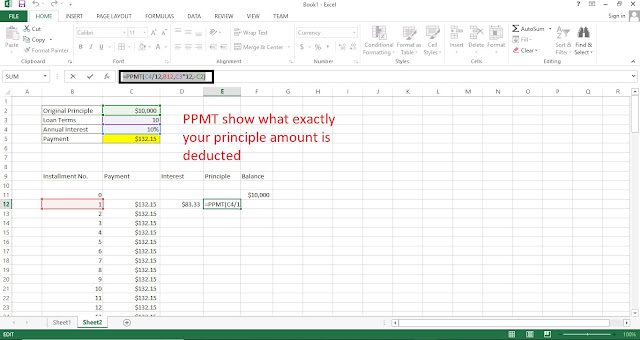### Recent

6/recent/ticker-posts

# Loan Amortization with Excel

Here in the first part, I am calculating the monthly payment i.e monthly installment payment for the loan. in the second part, I am going to calculate the number of installment with every month's interest, the principal amount paid, and the remaining balanceFunction Used

### PPMT

PMT(rate, nper, pv, [fv], [type])
Rate=The interest rate for our loan
nper=Number of installment i.e our total installment count
pv = Our principle value
[fv]=future value is optional
[type]= 0 or 1 also optional

IPMT(rate, per, nper, pv, [fv], [type])
Rate=The interest rate for our loan
nper=Number of installment i.e our total installment count
per=Installment number i.e which number of installment is calculating
pv = Our principle value
[fv]=future value is optional
[type]= 0 or 1 also optional

PPMT(rate, per, nper, pv, [fv], [type])

Rate=The interest rate for our loan
nper=Number of installment i.e our total installment count
per=Installment number i.e which number of installment is calculating
pv = Our principle value
[fv]=future value is optional
[type]= 0 or 1 also optional

Calculate the installment amount using PMT function
IN C5 Type =PMT(C4/12,C3*C6,-C2) (don't forget to give - for principal amount)

Calculate The Loan Amortization
first, give the number of payment series, you can calculate the number series by multiplying 12 with the loan terms or if your installment is not monthly
you can calculate multiplying terms with no of installment per year

=PMT(C4/12,C3*C6,-C2) just call =\$C\$5 don't forget to make cell absolute cell references

Calculate the interest amount using IPMT formulas
calculate interest amount using IPMT function of excel
=IPMT(\$C\$4/12,B12,\$C\$3*\$C\$6,-\$C\$2)

Calculte your paid  principle amount using PPMT function
=PPMT(\$C\$4/12,B12,\$C\$3*\$C\$6,-\$C\$2)Calculate remaining balance
The remaining balance is your principle minus paid principle

=F11-E12